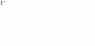# Nodal lines and single slit experiment

• Arnoldjavs3

## Homework Statement

The diagram above shows a single slit with lines marking the direction to a point P in the diffraction pattern. Two wavelets at x and y pass through the edges of the slit as shown. The possible value for path difference, py-px, that places P at the second nodal line next to the central maximum is?

## The Attempt at a Solution

I'm not sure if its (5λ)/2 or something else.
Just to clarify: Maximas are lcoated where a nodal line is formed(and of which a nodal line is just a line passing through points of constructive interference)
So if it is two nodal lines away from the central maximum, that would mean its at the third maximum starting from the first.
Am i correct?
(If it is only one nodal line, will it be 3λ/2? I think so.)

#### Attachments

•373 bytes · Views: 466

Is it possible to share the diagram? Also, nodal lines are areas of destructive interference and thus are minima.

I actually have an attachment if you can see it(I'd imagine it'd be a bit hard... but the diagram isn't really too useful)
Okay so I have had the wrong idea then. Would it be 1λ/2 and 2λ then?

Do you have the formula for the path difference?

Oh okay. The formula is
PD = (n
but the thing is they have to be out of phase(nodal line) for the minimum to appear. So minima appear where there are nodal lines present. Thus of which leads to being 1λ, 2λ, etc out of phase

Now, just replace your formula with the order the question is looking for (n=2) and you have it.

Last edited:
So that would suggest that I'm right? 2nd nodal line = 2lambda

Yessir!